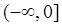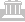Quality MaterialsSaving You Money

## Precalculus

Our precalculus textbook has a student workbook and lecture videos available.

###### Prerequisite help:

Section 1.1

Distance Formula

Exponents

More Exponents

More Exponents

Simplifying Expressions with Exponents

Polynomial Multiplication

Section 1.2

Section 1.3

Slope

Section 1.4

Section 1.5

Even and Odd Functions

Section 1.6

Section 2.1

Linear Equations

Section 2.2

Graphing Linear Equations

Section 2.3

Solving Literal Equations

Section 2.4

Section 2.5

Solving Absolute Value Equations

Section 3.1

Polynomials

Section 3.2

Section 3.3

Section 3.4

Polynomial Long Division

Synthetic Division

Section 3.5

Section 3.6

Complex Numbers

Section 3.7

Simplifying Rational Expressions

Section 3.8

Section 4.1

###### Alternate Explanations:

Section 1.1

Function Notation

Functions

Section 1.2

Domain and Range

Piecewise Functions

Section 1.3

Section 1.4

Composition of Functions

Section 1.5

Function Transformations

Section 1.6

Inverse Functions

Section 2.1

Section2.2

Section2.3

Section 2.4

Section 2.5

Solving Absolute Value Inequalities

Section 3.1

Section 3.2

Completing the Square to Find the Vertex

Section 3.3

Polynomial Graphs

Solving Polynomial Inequalities

Finding Polynomials From Their Zeros

Section 3.4

Factor Theorem

Section 3.5

Solving Polynomials

Rational Roots Test

Section 3.6

Section 3.7

Graphing Rational Equations

Asymptotes

Section 3.8

Section 4.1

Exponential Functions

Section 4.2

Graphing Exponential Functions

Section 4.3

Logarithms

Section 4.4

Logarithmic Properties

Section 4.5

Graphing Logarithmic Functions

Section 4.6

Solving Exponential Equations

Examples of Word Problems

Corrections in Textbook:

pg 135 problem 5 part a.

1. Find a formula for the moose population, P, t years after 1990.

2. pg 135 problem 6 part a

1. Find a formula for the owl population, P, t years after 2000.

3. pg 162 34. k(n) not k(u).

Corrections in answers in back of textbook:

Section 2.1 #25 mph should be miles per minute.

Section 3.2 #39. a.b. 632812.5 ft   c. 56125 ft    d. 1.11ft

Section 3.3 #25.Section 3.4 #1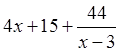Section 3.4 #11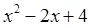Section 3.4 #17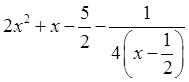Section 3.4 #21 1,2,3

Section 3.5 #19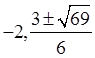Section 3.6 #27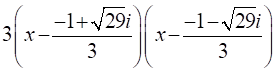Section 3.6 #29 Zeros: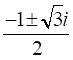Section 3.6 #35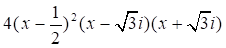Section 3.6 #39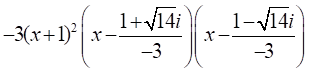Section 3.7 #13 oblique should be 2x+9 not 2x.

Section 3.8 #1 Domain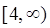Section 3.8 #3 Domain